# pylops.Restriction¶

class pylops.Restriction(M, iava, dims=None, dir=0, dtype='float64', inplace=True)[source]

Restriction (or sampling) operator.

Extract subset of values from input vector at locations iava in forward mode and place those values at locations iava in an otherwise zero vector in adjoint mode.

Parameters: M : int Number of samples in model. iava : Integer indices of available samples for data selection. dims : list Number of samples for each dimension (None if only one dimension is available) dir : int, optional Direction along which restriction is applied. dtype : str, optional Type of elements in input array. inplace : bool, optional Work inplace (True) or make a new copy (False). By default, data is a reference to the model (in forward) and model is a reference to the data (in adjoint).

pylops.signalprocessing.Interp
Interpolation operator

Notes

Extraction (or sampling) of a subset of $$N$$ values at locations iava from an input (or model) vector $$\mathbf{x}$$ of size $$M$$ can be expressed as:

$y_i = x_{l_i} \quad \forall i=1,2,...,M$

where $$\mathbf{l}=[l_1, l_2,..., l_M]$$ is a vector containing the indeces of the original array at which samples are taken.

Conversely, in adjoint mode the available values in the data vector $$\mathbf{y}$$ are placed at locations $$\mathbf{l}=[l_1, l_2,..., l_M]$$ in the model vector:

$x_{l_i} = y_i \quad \forall i=1,2,...,M$

and $$x_{j}=0 j \neq l_i$$ (i.e., at all other locations in input vector).

Attributes: shape : tuple Operator shape explicit : bool Operator contains a matrix that can be solved explicitly (True) or not (False)

Methods

 __init__(self, M, iava[, dims, dir, dtype, …]) Initialize this LinearOperator. adjoint(self) Hermitian adjoint. cond(self, \*\*kwargs_eig) Condition number of linear operator. conj(self) Complex conjugate operator div(self, y[, niter]) Solve the linear problem $$\mathbf{y}=\mathbf{A}\mathbf{x}$$. dot(self, x) Matrix-matrix or matrix-vector multiplication. eigs(self[, neigs, symmetric, niter]) Most significant eigenvalues of linear operator. mask(self, x) Apply mask to input signal returning a signal of same size with values at iava locations and 0 at other locations matmat(self, X) Matrix-matrix multiplication. matvec(self, x) Matrix-vector multiplication. rmatvec(self, x) Adjoint matrix-vector multiplication. transpose(self) Transpose this linear operator.
mask(self, x)[source]

Apply mask to input signal returning a signal of same size with values at iava locations and 0 at other locations

Parameters: x : numpy.ndarray Input array (can be either flattened or not) y : numpy.ma.core.MaskedArray Masked array.

## Examples using pylops.Restriction¶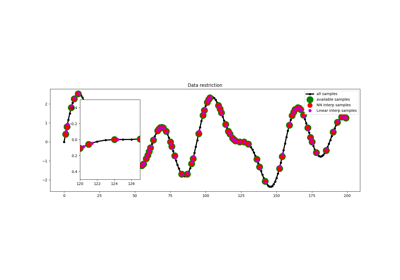Restriction and Interpolation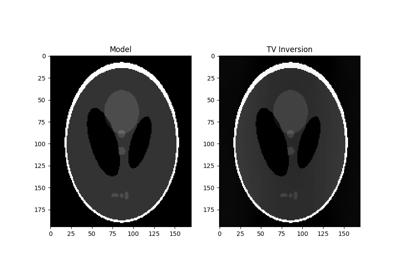Total Variation (TV) Regularization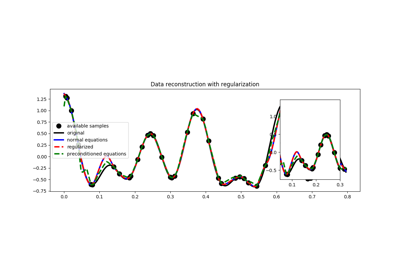03. Solvers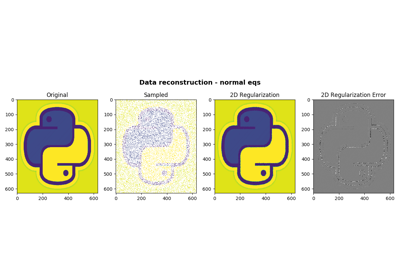05. 2D Interpolation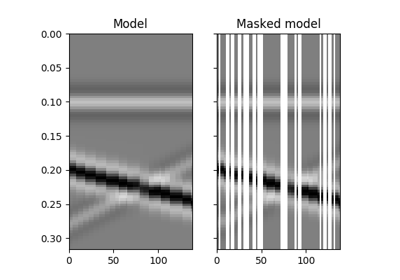11. Seismic regularization